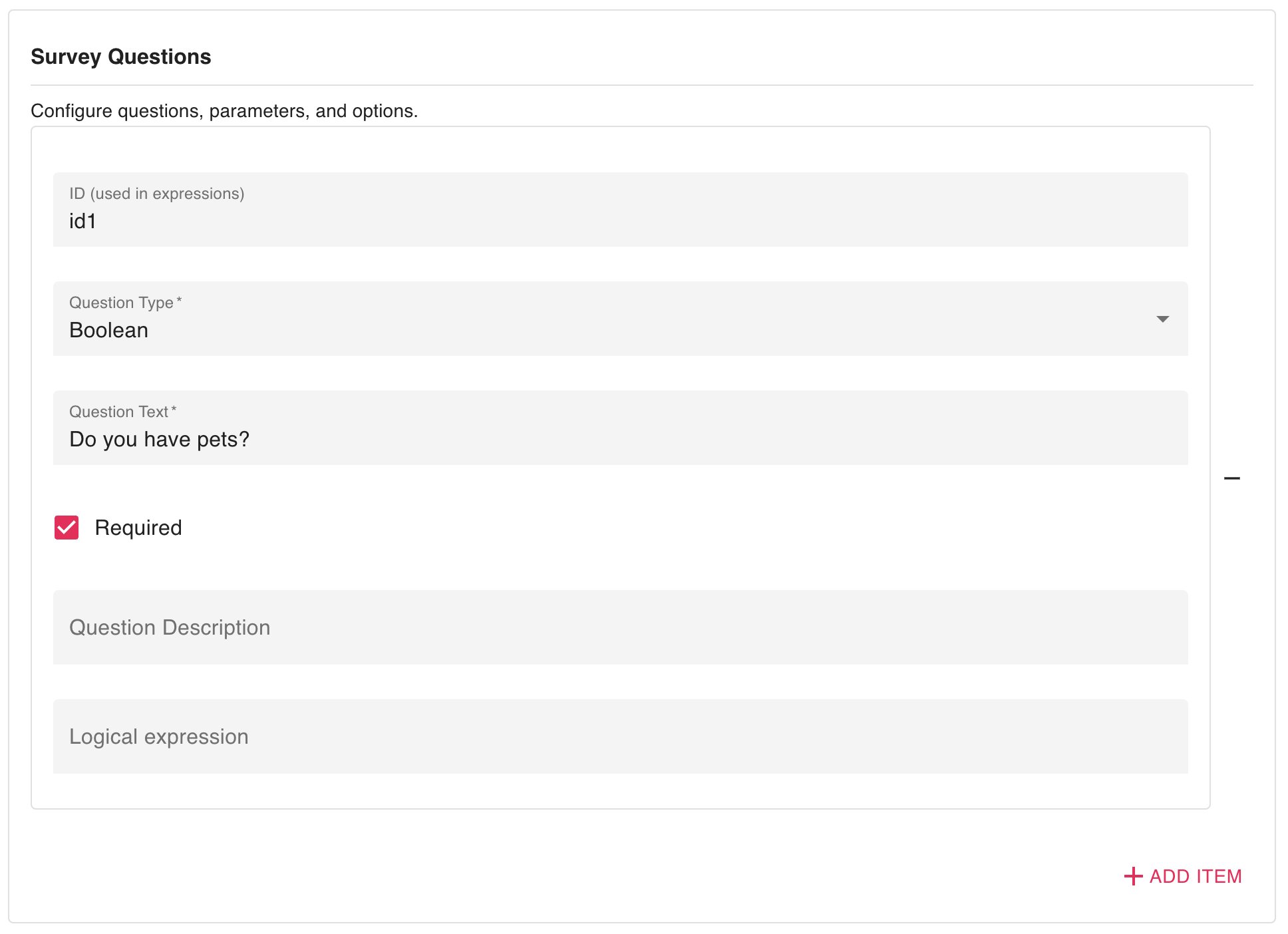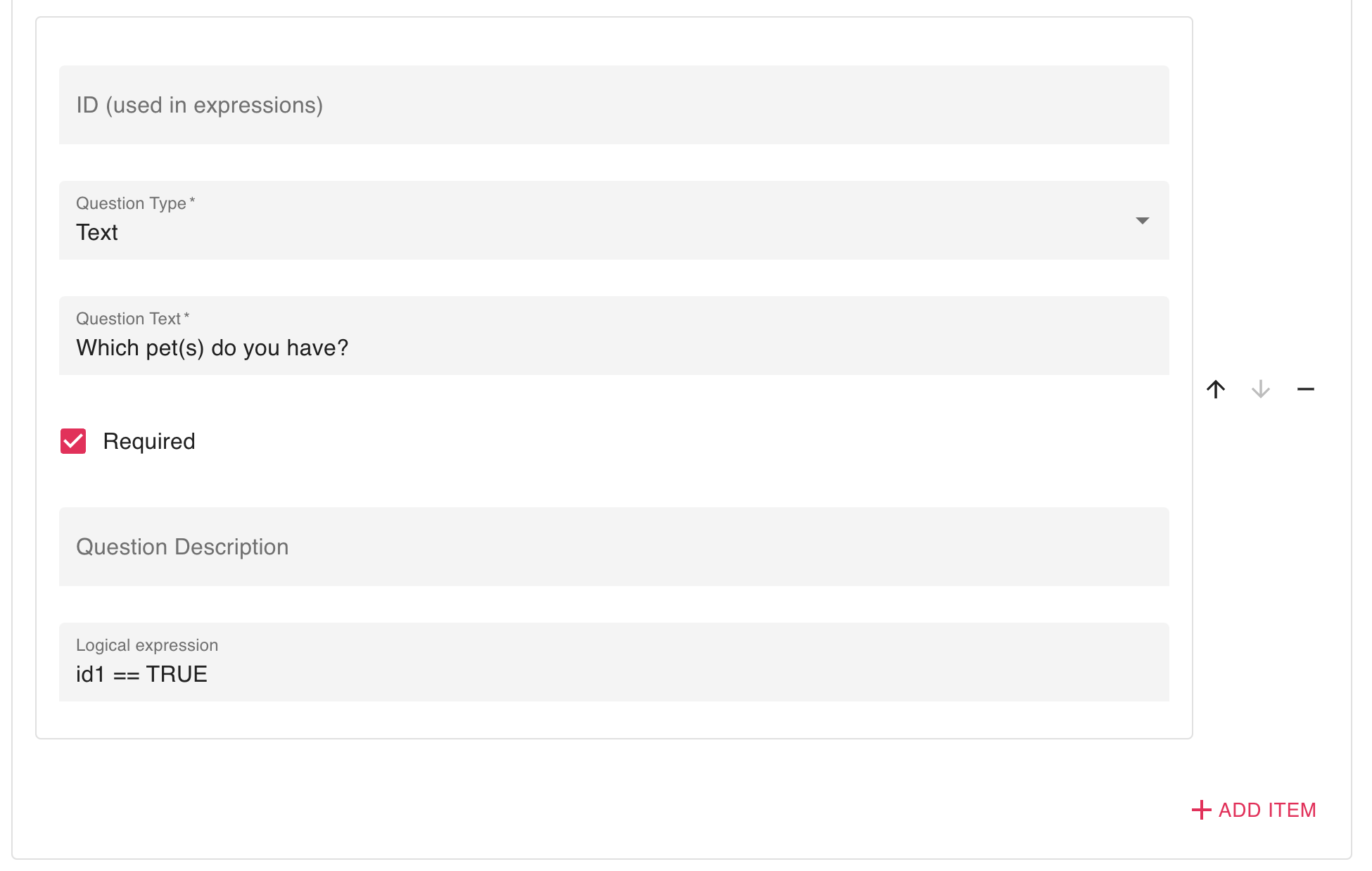Professionals can specify expressions on survey questions. An expression will determine whether the survey question will be shown to the participant or not.

## 5.1 Instructions

As of now, expressions based on the following question types are supported:

• Boolean
• List
• Multi-Select
• Slider
• Rating
• Time
• Likert

### 5.1.1 Syntax

Expressions must be of the format:

ID OPERATOR VALUE

And ID can be any specified question identifier (see 5.2 Example).

OPERATOR is one of the following:

• ==
• !=
• >
• <
• >=
• <=
• MATCHES

Where VALUE is either TRUE, FALSE, numeric value, an answer ID, a regex pattern or a timestamp. See below for more information.

Note that the >, < >= and <= are only compatible with numeric or timestamp values.

Expressions can be combined with AND and OR operators, for example:

id1 == TRUE OR id2 <= 5 AND A === b

### 5.1.2 Possible value combinations

#### 5.1.2.1 Boolean

Boolean values, i.e. TRUE or FALSE, can be combined with the following question types:

• Boolean

Example:

A == TRUE

#### 5.1.2.2 Numeric

Numeric values can be combined with the following question types:

• Rating
• Slider
• Likert, where:
• 0 = “Not at all”
• 1 = “Several Times”
• 2 = “More than Half the Time”
• 3 = “Nearly All the Time”

Example:

A <= 3

Variable values can be combined with the following question types:

• List
• Multi-Select

Note: be sure to set variable names to the answers you’ve specified.

Example:

A == a

#### 5.1.2.4 Regex pattern

Regex values can be combined with the following question types:

• Text

Note: regex is only compatible with the MATCHES operator

Example:

A MATCHES /dog/gi

#### 5.1.2.5 Timestamp

Regex values can be combined with the following question types:

• Time

Note: all operators, except MATCHES, are compatible with timestamp values.

Example:

A >= 21:00 OR A < 9:30

## 5.2 Expression configuration example

While creating a Survey activity:

1. First create a question of any of the allowed Question Type.1. Give this question an “ID” and a “Question Text”.